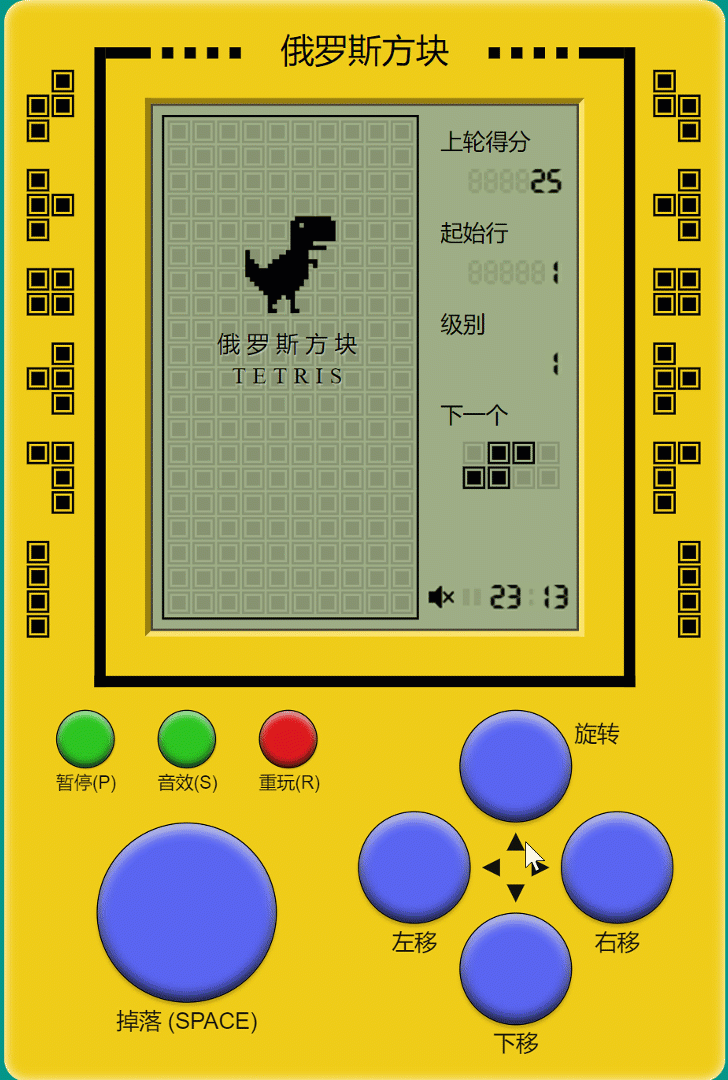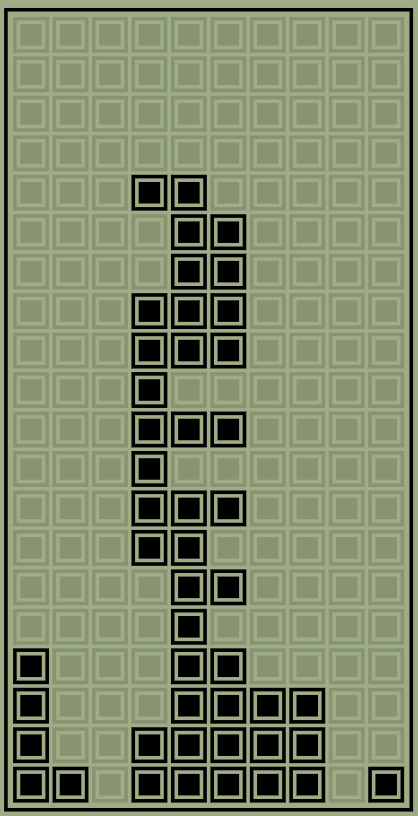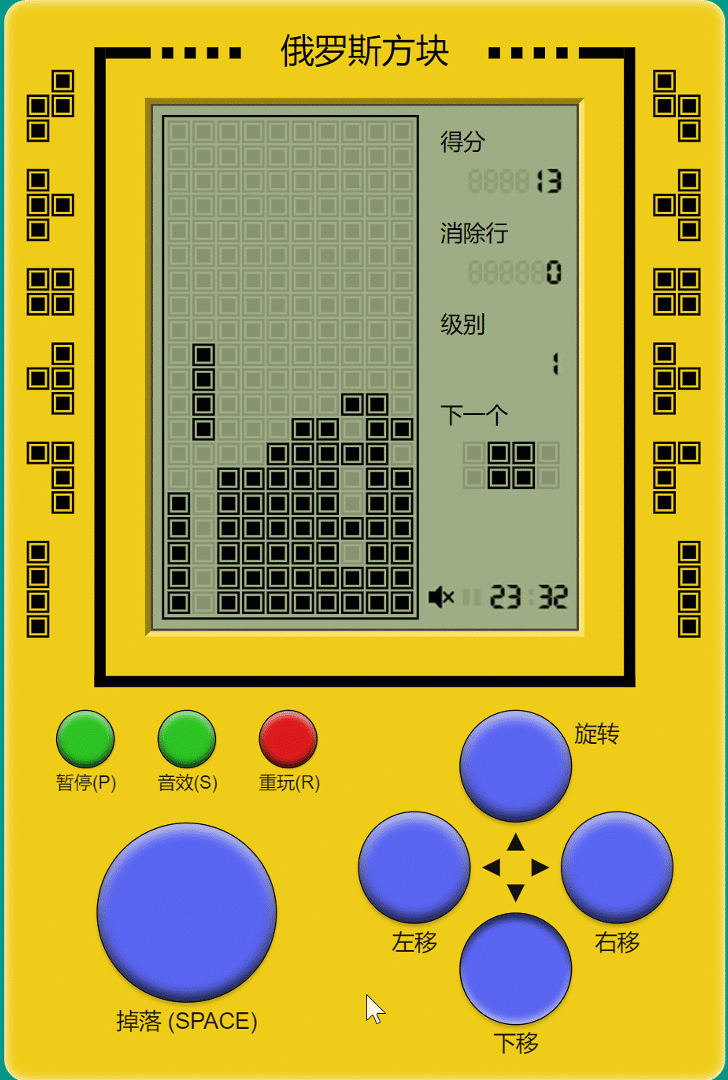# 案例手把手带你从零开始完整开发经典游戏【俄罗斯方块】，全部逻辑只用不到200行代码。

### 最终效果演示### 游戏规则

1. 有一个用于摆放小型正方形的场地：宽10，高20。

2. 一组由4个小型正方形组成的规则图形，共有7种，分别以S、Z、L、J、I、O、T这7个字母的形状来命名。

3. 场地顶部随机地产生方块，以一定的规则进行移动、旋转、下落和摆放，锁定并填充到场地中。每次摆放如果将场地的一行或多行完全填满，则组成这些行的所有小正方形将被消除，并奖励一定的积分。而未被消除的方块会一直累积，并对后来的方块摆放造成各种影响。

4. 随着积分的增加，方块的掉落速度也会逐渐增加，提升游戏难度和趣味性。

5. 方块移到区域最下方或是落到其他方块上无法移动时，就会固定在该处。未被消除的方块堆放的高度超过场地所规定的最大高度时，游戏结束。

### 用二维数组矩阵控制场地的渲染

``````[
[0, 0, 0, 0, 0, 0, 0, 0, 0, 0],
[0, 0, 0, 0, 0, 0, 0, 0, 0, 0],
[0, 0, 0, 0, 0, 0, 0, 0, 0, 0],
[0, 0, 0, 0, 0, 0, 0, 0, 0, 0],
[0, 0, 0, 1, 1, 0, 0, 0, 0, 0],
[0, 0, 0, 0, 1, 1, 0, 0, 0, 0],
[0, 0, 0, 0, 1, 1, 0, 0, 0, 0],
[0, 0, 0, 1, 1, 1, 0, 0, 0, 0],
[0, 0, 0, 1, 1, 1, 0, 0, 0, 0],
[0, 0, 0, 1, 0, 0, 0, 0, 0, 0],
[0, 0, 0, 1, 1, 1, 0, 0, 0, 0],
[0, 0, 0, 1, 0, 0, 0, 0, 0, 0],
[0, 0, 0, 1, 1, 1, 0, 0, 0, 0],
[0, 0, 0, 1, 1, 0, 0, 0, 0, 0],
[0, 0, 0, 0, 1, 1, 0, 0, 0, 0],
[0, 0, 0, 0, 1, 0, 0, 0, 0, 0],
[1, 0, 0, 0, 1, 1, 0, 0, 0, 0],
[1, 0, 0, 0, 1, 1, 1, 1, 0, 0],
[1, 0, 0, 1, 1, 1, 1, 1, 0, 0],
[1, 1, 0, 1, 1, 1, 1, 1, 0, 1]
]``````### 7种俄罗斯方块的形状

``````[
[
[0, 0, 0, 0],
[1, 1, 1, 1]
],
[
[0, 0, 1, 0],
[1, 1, 1, 0]
],
[
[1, 0, 0, 0],
[1, 1, 1, 0]
],
[
[1, 1, 0, 0],
[0, 1, 1, 0]
],
[
[0, 1, 1, 0],
[1, 1, 0, 0]
],
[
[0, 1, 1, 0],
[0, 1, 1, 0]
],
[
[0, 1, 0, 0],
[1, 1, 1, 0]
]
]``````

### 键盘操控和点击操控

``````{
"32": "drop",
"37": "moveLeft",
"38": "rotate",
"39": "moveRight",
"40": "down",
"80": "pause",
"82": "replay",
"83": "sound"
}``````

``````\$v.activeKey = \$event.keyCode
\$c.exp[\$v.KEYS[\$v.activeKey]].exc()``````

activeKey表示当前按下的按键，\$v.KEYS[\$v.activeKey]是上面绑定的要执行的动作名称，这些动作的定义都放在\$c.exp中，会在下面的篇幅种逐个讲解。

``````\$v.activeKey = undefined
\$(".key > .active").removeClass("active")``````

### 游戏开始：start

``````\$v.score = 0
\$v.status = "fall"
\$v.startline ? array(\$v.startline).forEach(\$c.exp.startline) : ""
\$c.exp.next.exc()``````

### 初始化起始行：startline

``````\$v.matrix[19 - \$index] = array(10,1)
array(1 + \$w.Math.floor(\$w.Math.random() * 5)).forEach('\$v.matrix[19 - \$ext.\$index][\$w.Math.floor(\$w.Math.random() * 9)] = 0')``````

### 产生下一个俄罗斯方块：next

``````\$v.currB = \$v.nextB
\$v.nextB = \$v.blocks[\$w.Math.floor(\$w.Math.random() * \$v.blocks.length)]
\$v.xy = [3, -1]
\$c.exp.fall.exc()``````

`\$v.blocks`里随机产生一个俄罗斯方块（currB表示当前方块current Block，nextB表示下一个方块next Block），并把它的位置设在最顶部（x起点为3，中间位置，y起点在-1，也就是还在矩阵外，看不见）。

### 开始下落：fall

``````stopIf(\$v.status !== "fall" || (\$l.fall && \$l.fall !== \$v.fall))
\$c.exp.meetIf.exc()
\$v.xy = \$v.xy + 1
render()
timeout((10 - \$v.level) * (\$v.activeKey ? 200 : 100))
\$c.exp.fall.exc()``````

### 碰撞检查：meetIf

``````stopIf(\$v.status != "fall" || !\$v.currB.length)
stopIf(\$v.currB.some('\$x.some("\$x && \$v.matrix[\$ext.\$index + \$v.xy + 1][\$index + \$v.xy] !== 0")'), \$c.exp.meet)``````

### 发生碰撞了：meet

``````stopIf(\$v.xy === -1, \$c.exp.replay)
\$v.currB.forEach('\$x.forEach("\$x ? \$c.exp.meet2.exc() : null")')
\$v.currB = []
render()
timeout(300)
\$\$(".matrix .red").removeClass("red")
timeout(300)
\$v.score = (\$v.score || 0) + \$v.level
\$l.clearY = []
\$v.matrix.forEach('\$x.includes(0) ? "" : \$l.clearY.push(\$index)')
\$l.clearY.length ? \$c.exp.clearline.exc() : timeout(100)
\$c.exp.next.exc()``````

### 碰撞动作：meet2

``````\$v.matrix[\$ext.\$index + \$v.xy][\$index + \$v.xy] = 1
\$v.el.matrix.children[\$ext.\$index + \$v.xy].children[\$index + \$v.xy].addClass("red")``````

### 消行：clearline

``````\$l.clearY.forEach('\$v.el.matrix.children[\$x].addClass("red")')
timeout(300)
\$l.clearY.forEach('\$v.el.matrix.children[\$x].removeClass("red")')
timeout(310)
\$l.clearY.forEach('\$v.matrix.splice(\$x, 1); \$v.matrix.splice(0, 0, array(10, 0))')
\$v.score = (\$v.score || 0) + \$w.Math.pow(2, \$l.clearY.length) * \$v.level * 10
\$v.lines = (\$v.lines || 0) + \$l.clearY.length``````### 左移：moveLeft

``````\$l.lr = -1
\$c.exp.moveLR.exc()``````

### 右移：moveRight

``````\$l.lr = 1
\$c.exp.moveLR.exc()``````

### 移动逻辑：moveLR

``````stopIf(!\$v.activeKey || \$v.currB.some('\$x.some("\$x && \$v.matrix[\$ext.\$index + \$v.xy][\$index + \$v.xy + \$l.lr] !== 0")'))
stopIf(\$v.status === "paused", '\$v.status = "fall"; ' + \$c.exp.fall)
\$v.xy = \$v.xy + \$l.lr
render()
timeout(150 - \$v.level * 10)
\$c.exp.moveLR.exc()``````

### 旋转：rotate

``````\$l.currB = []
\$v.currB.length === 2 ? \$c.exp.rotate2to4.exc() :  \$c.exp.rotate4to2.exc()
stopIf(\$l.currB.some('\$x.some("\$x && \$v.matrix[\$ext.\$index + \$l.xy][\$index + \$l.xy] !== 0")'))
\$v.currB = \$l.currB
\$v.xy = \$l.xy``````

### 竖起来：rotate2to4

``````array(4).forEach('\$l.currB.push([\$v.currB[\$index], \$v.currB[\$index]])')
\$l.xy = [\$v.xy + 1, \$v.xy - 1]``````

### 横躺：rotate4to2

``````array(2).forEach('\$l.currB.push([\$v.currB[\$index], \$v.currB[\$index], \$v.currB[\$index], \$v.currB[\$index]])')
\$l.xy = [\$v.xy - 1, \$v.xy + 1]``````

### 开始速降：down

``````stopIf(\$v.status === "paused", '\$v.status = "fall"; ' + \$c.exp.fall)
\$v.fall = date()
\$l.fall = \$v.fall
\$c.exp.downLoop.exc()``````

### 速降逻辑：downLoop

``````\$c.exp.meetIf.exc()
\$v.xy = \$v.xy + 1
render()
timeout(20 - \$v.level)
stopIf(\$v.activeKey, \$c.exp.downLoop)
\$c.exp.fall.exc()``````

### 开始掉落：drop

``````stopIf(\$v.status === "over")
stopIf(\$v.status === "ready", \$c.exp.start)
stopIf(\$v.status === "paused", '\$v.status = "fall"; ' + \$c.exp.fall)
\$v.fall = date()
timeout(9)
\$v.el.top.removeClass("dropping")
\$c.exp.dropLoop.exc()``````

over了也就不掉落了。

### 掉落逻辑：dropLoop

``````\$c.exp.meetIf.exc()
\$v.xy = \$v.xy + 1
\$c.exp.dropLoop.exc()``````

### 暂停游戏：pause

``````stopIf(\$v.status === "over")
stopIf(\$v.status === "ready", \$c.exp.start)
stopIf(\$v.status === "fall", '\$v.status = "paused"; ' + \$c.exp.pauseAnim)
\$v.status = "fall"
\$c.exp.fall.exc()``````

over了也就没必要暂停了。

### 暂停动画：pauseAnim

``````toggleClass(\$v.el.paused, "c")
timeout(300)
\$v.status === "paused" ? \$c.exp.pauseAnim.exc() : ""``````

### 开关音效：sound

``\$v.music = !\$v.music``

``\$v.music && \$audio.start(when, offset, duration)``

### 重玩：replay

``````stopIf(\$v.status === "ready", \$c.exp.start)
\$v.currB = []
\$v.hiscore = \$w.Math.max(\$v.hiscore, \$v.score)
\$v.lines = 0
\$v.matrix = array(20, array(10))
\$v.status = "over"
render()
array(20).forEach('\$v.el.matrix.children[19 - \$index].addClass("c"); timeout(30)')
timeout(100)
array(20).forEach('\$v.el.matrix.children[\$index].removeClass("c"); timeout(30)')
render()

``````timeout(1000)
\$l.dragon = \$(".dragon")
stopIf(!\$l.dragon)
\$l.dragonClass = "r"
timeout(1000)
\$l.dragonClass = "l"
\$v.status === "ready" ? \$c.exp.readyAnim.exc() : ""``````

``````\$l.dragon.className = "dragon " + \$l.dragonClass + (\$index + 1) % 4
timeout(60)``````

### 场景保存/复原：visibilitychange

``\$w.document.addEventListener('visibilitychange', func('\$w.document.visibilityState === "hidden" ? \$exp.save.exc() : \$exp.restore.exc()'))``

### 保存状态：save

``````localStorage("tetris", {music: \$v.music, startline: \$v.startline, level: \$v.level, score: \$v.score, lines: \$v.lines, currB: \$v.currB, nextB: \$v.nextB, status: \$v.status === "over" ? "ready" : \$v.status, hiscore: \$w.Math.max(\$v.hiscore, \$v.score), matrix: \$v.status === "over" ? array(20, array(10)) : \$v.matrix, xy: \$v.xy > 19 ? [3, -1] : \$v.xy})
\$v.status === "fall" ? \$v.status = "paused" : ""``````

### 复原状态：restore

``````\$l.LS = localStorage("tetris")
\$v.score = \$l.LS.score || 0
...
render()
\$v.status === "fall" ? exc('timeout(2000); ' + \$c.exp.fall) : ""``````

• 怎样获取键盘输入？

• 怎样控制方块的移动？

• 游戏中的各种形状及整个游戏空间怎么用数据表示？

• 游戏中怎么判断左右及向下移动的可能性？

• 游戏中怎么判断某一形状旋转的可能性？

• 按向下方向键时加速某一形状下落速度的处理？

• 怎么判断某一形状已经到底？

• 怎么判断某一行已经被填满？

• 怎么消去某一形状落到底后能够消去的所有的行？

• 怎样修改游戏板的状态？

• 怎样统计分数？

• 怎样处理升级后的加速问题？

• 怎样判断游戏结束？